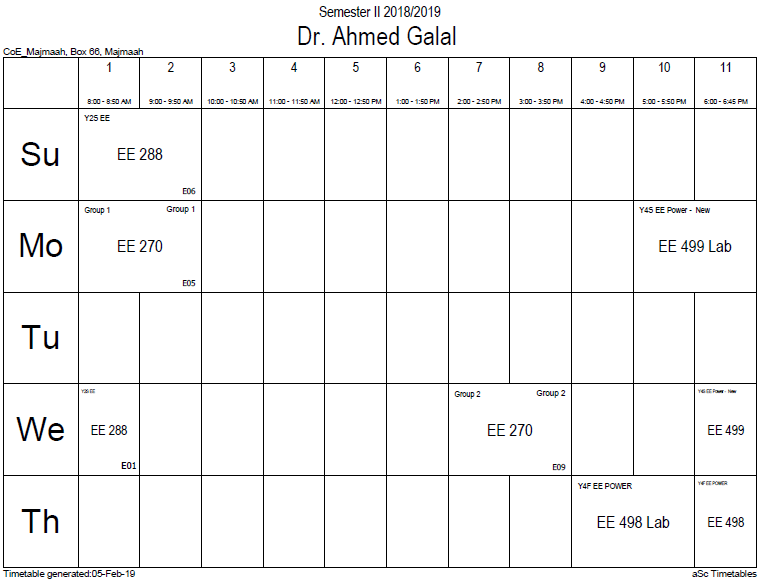## Force between magn

The force between two small magnets is quite complicated and depends on the strength and orientation of both magnets and the distance and direction of the magnets relative to each other. The force is particularly sensitive to rotations of the magnets due to magnetic torque. The force on each magnet depends on its magnetic moment and the magnetic field of the other.

To understand the force between magnets, it is useful to examine the magnetic pole model given above. In this model, the H-field of one magnet pushes and pulls on both poles of a second magnet. If this H-field is the same at both poles of the second magnet then there is no net force on that magnet since the force is opposite for opposite poles. If, however, the magnetic field of the first magnet is nonuniform (such as the H near one of its poles), each pole of the second magnet sees a different field and is subject to a different force. This difference in the two forces moves the magnet in the direction of increasing magnetic field and may also cause a net torque.

This is a specific example of a general rule that magnets are attracted (or repulsed depending on the orientation of the magnet) into regions of higher magnetic field. Any non-uniform magnetic field whether caused by permanent magnets or by electric currents will exert a force on a small magnet in this way.

The details of the Amperian loop model are different and more complicated but yield the same result: that magnetic dipoles are attracted/repelled into regions of higher magnetic field. Mathematically, the force on a small magnet having a magnetic moment m due to a magnetic field B is:$mathbf{F} = mathbf{ abla} left(mathbf{m}cdotmathbf{B} ight),$

where the gradient is the change of the quantity m · B per unit distance and the direction is that of maximum increase of m · B. To understand this equation, note that the dot product m · B = mBcos(θ), where m and B represent the magnitude of the m and B vectors and θ is the angle between them. If m is in the same direction as B then the dot product is positive and the gradient points 'uphill' pulling the magnet into regions of higher B-field (more strictly larger m · B). This equation is strictly only valid for magnets of zero size, but is often a good approximation for not too large magnets. The magnetic force on larger magnets is determined by dividing them into smaller regions having their own m then summing up the forces on each of these regions.

### Office HoursNo office hours### My Timetable### Contactsemail: [email protected]

Phone: 2570

### Welcome

Welcome To Faculty of Engineering### IEEEhttp://www.ieee.org/

/

### Bookmarkshttp://www.utk.edu/research/

http://science.doe.gov/grants/index.asp

http://www1.eere.energy.gov/vehiclesandfuels/

http://www.eere.energy.gov/

### Upcoming Conferences### Engineering quotes### Travel Web Siteshttp://www.hotels.com/

http://www.orbitz.com/

http://www.hotwire.com/us/index.jsp

http://www.kayak.com/

### Blackboardستقام اختبارات الميدتيرم يوم الثلاثاء 26-6-1440

حسب الجدول المعلن بلوحات الاعلان

### Summer trainingThe registration for summer training will start from 5th week of second semesterClass registration week 1

### برنامج التجسير### إحصائية الموقع

عدد الصفحات: 2879

البحوث والمحاضرات: 1280

الزيارات: 101050